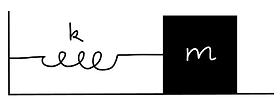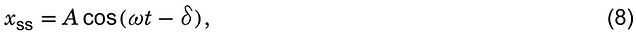top of page

## The equation of motionNewton's equation for a harmonic oscillator with mass m, damping coefficient β, spring constant k under force f(t) isDiving Eq. (1) by and rearranging,whereEq. (2) can easily be solved using MATLAB. Let us first defined a state vectorThen, it follows thatIn MATLAB, Eq. (5), which express the derivative of x in terms of x, is defined by a function:

function dx = oscillator(t, x, omega_0, beta, g)
dx = zeros(2,1);
% a column vector
dx(1) = x(2);
dx(2) = -2*beta*x(2)-omega_0^2*x(1)+g;

Copy and paste the above code, and save it as oscillator.m. Now, let us numerically observe how the harmonic oscillator behaves under three different types of g(t).

## 1. Unforced oscillations

Unforced oscillations mean g(t) = 0. Let us assume that ω0 = 2π, β = 0, and the following initial condition:Copy and paste the following code, and save it as, for example, SHO_unforced.m.

omega_0 = 2*pi;
beta = 0.0;
t_max = 10;
% the simulation runs for 0 <= t <= t_max
x1_0 = 1; % initial condition: x(0)=0
x2_0 = 0; % initial contition: x'(0)=0
[T,X] = ode45(@(t,x)oscillator(t, x, omega_0, beta, 0), [0 t_max], [x1_0 x2_0]);

plot(T, X(:,1))
xlabel('time')
ylabel('x(t)')

Place oscillator.m and SHO_unforced.m into a folder, and run SHO_unforced.m.

Is the obtained x(t) consistent with the initial condition? What is the frequency of the oscillation?

Repeat the simulation with different values of β, and see how x(t) is influnced by β.

## 2. Forced oscillations: step driving force

In this case, g(t) = u(t), where u(t) is the step function:Copy and paste the following code, and save it as, for example, SHO_forced_step.m.

omega_0=2*pi;
beta=0.5;
t_max = 20;
% the simulation runs for 0 <= t <= t_max
x1_0 = 0; % initial condition: x(0) = 0.
x2_0 = 0.0; % initial condition: x'(0) = 0.
[T,X] = ode45(@(t,x)oscillator(t,x, omega_0,beta, 1), [0 t_max], [x1_0 x2_0]);

plot(T, X(:,1)),
xlabel('time')
ylabel('x(t)')

Run SHO_forced_step.m. What is the steady-state value of x(t)? Is it equal to the steady-state value that can be calculated from Eq. (2). What is the frequency of the initial oscillations (the transient response for this case) observed in early time? How does β affect the time taken for x(t) to reach the steady state value?

## 3. Forced oscillations: sinusoidal driving force

In this case, g(t) = cosωt u(t). Copy and paste the following code, and save it as, for example, SHO_forced_sinusoidal.m.

omega_0=2*pi;
beta=0.1;
omega =1;
% frequency of g(t)

t_max = 2*pi/omega*15; % the simulation runs for 0 <= t <= t_max
x1_0 = 0; % initial condition x(0) = 0.
x2_0 = 0.0; % initial condition x'(0) = 0.
[T, X] = ode45(@(t,x)oscillator(t, x, omega_0, beta, cos(omega*t)), [0 t_max], [x1_0 x2_0]);

h1 = plot(T, X(:,1), 'linewidth', 2),
hold on;
plot(T, cos(omega*T)*max(X(:,1)), 'r', 'linewidth', 2)
grid on;
h2 = legend('x(t)', 'g(t)')
h2.FontSize = 15;
xlabel('time', 'fontsize', 15)
ax = ancestor(h1, 'axes')
ax.XAxis.FontSize = 15;
ax.YAxis.FontSize = 15;

Run SHO_forced_sinusoidal.m for different values of ω and β. Interestingly, and importantly, you will see that the steady-state x(t) is also sinusoidal with an angular frequency ω, which is equal to the angular frequency of g(t). For example, x(t) for ω0 = 2π, β = 0.1 and ω = 1 is shown below, along with g(t).For different values of β, plot the amplitude and the phase of the steady-state x(t) versus ω. That is, when the steady-state x(t) is written asplot A and δ versus ω.

bottom of page